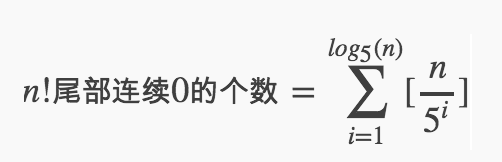@Yano 2019-09-20T02:54:09.000000Z 字数 2896 阅读 1577

# LeetCode 数字 题目汇总

LeetCode

# 公众号

coding 笔记、点滴记录，以后的文章也会同步到公众号（Coding Insight）中，希望大家关注^_^

https://github.com/LjyYano/Thinking_in_Java_MindMapping# 7. Reverse Integer

Reverse digits of an integer.

Example1: x = 123, return 321
Example2: x = -123, return -321

click to show spoilers.

Note:
The input is assumed to be a 32-bit signed integer. Your function should return 0 when the reversed integer overflows.

• 负数
• 溢出

## 代码

public class Solution {    public int reverse(int x) {        long ans = 0;        while(x != 0) {            ans = ans * 10 + x % 10;            x /= 10;            if(ans > Integer.MAX_VALUE || ans < Integer.MIN_VALUE)                return 0;        }        return (int)ans;    }}

# 231. Power of Two

Given an integer, write a function to determine if it is a power of two.

## 思路

• 可以根据 (n & (n - 1)) == 0 来判断，这是因为2的幂仅有1位是1，其余位全部是0；如果-1，那么1的那位会变成0，后面的位全部为1。
• 可以根据 (n & -n) == n 来判断，这是 JDK 中 Ingeger 中使用的判断方法。因为 -n 的二进制表示，恰好相当于（n - 1）取反，而符号位本来就是不同的。本质上和上一种解放是一样的。

## 代码

public class Solution {    public boolean isPowerOfTwo(int n) {        if(n <= 0) return false;        return (n & (n - 1)) == 0;    }}

public class Solution {    public boolean isPowerOfTwo(int n) {        if(n <= 0) return false;        return (n & -n) == n;    }}

# 326. Power of Three

Given an integer, write a function to determine if it is a power of three.

Could you do it without using any loop / recursion?

## 代码

public class Solution {    public bool IsPowerOfThree(int n) {        // 1162261467 是小于 Integer.MAX_VALUE 的3的最大幂数        return n > 0 && (1162261467 % n == 0);    }}

# 342. Power of Four

Given an integer (signed 32 bits), write a function to check whether it is a power of 4.

Example:
Given num = 16, return true. Given num = 5, return false.

Follow up: Could you solve it without loops/recursion?

## 思路

• 0000 0001 = 1，是4的幂
• 0000 0010 = 2，不是4的幂
• 0000 0100 = 4，是4的幂

## 代码

public class Solution {    public boolean isPowerOfFour(int n) {        if(n <= 0) return false;        return ((n & (n - 1)) == 0) && ((n & 0x55555555) != 0);    }}

# 172. Factorial Trailing Zeroes

Given an integer n, return the number of trailing zeroes in n!.

Note: Your solution should be in logarithmic time complexity.

## 分析

30!结果转换成3进制，结尾有多少个连续的0？


3， 6， 9， 12， 15 ，18， 21， 24， 27， 30


3 = 3 * 1
6 = 3 * 2
12 = 3 * 4
15 = 3 * 5
21 = 3 * 7
24 = 3 * 8
30 = 3 * 10


9 = 3 * 3
18 = 3 * 3 * 2


27 = 3 * 3 * 3


30/3+30/9+30/27所代表的，就是最终结果。

30/9则计算了一次9、18、27，但是27中还有一个因子没有算。

30/27计算了一次27，至此，所有的因子都计算完毕。

30/3+30/9+30/27=10+3+1=14


n!中，结尾有多少个连续的0


100！中，结尾有多少个连续的0？

100/5 + 100/25 + 100/125 = 20 + 4 + 0 = 24


## 计算公式public int trailingZeroes2(int n) {    int rt = 0;    for (int i = 5; i <= n; i *= 5) {        rt += n / i;    }    return rt;}

## 代码

public class Solution {    public int trailingZeroes(int n) {        int rt = 0;        for (long i = 5; i <= n; i *= 5) {            rt += n / i;        }        return rt;    }}

# 总结• 私有
• 公开
• 删除